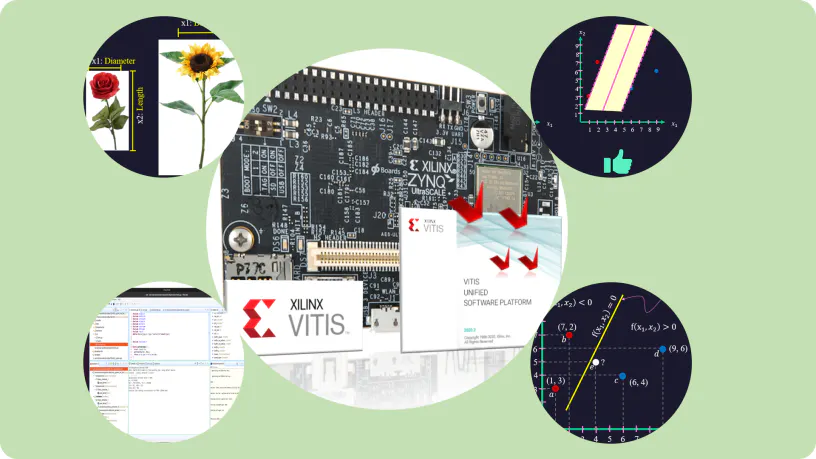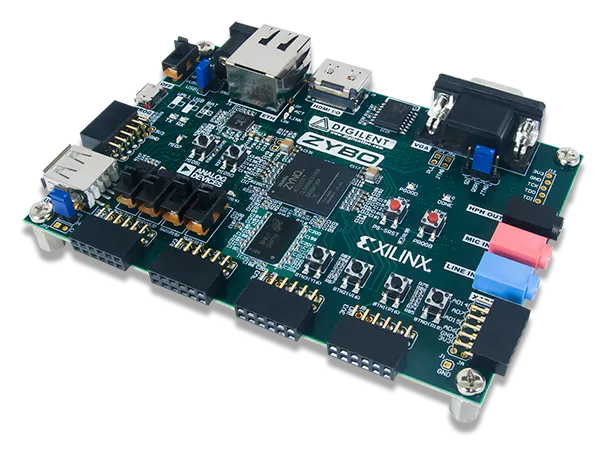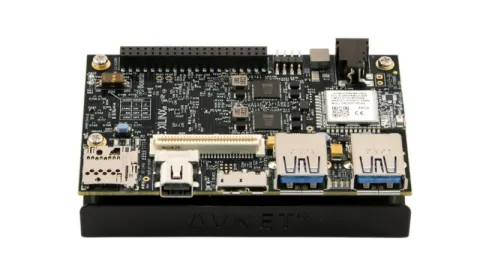# Support Vector Machine on Zynq & ZynqMPSoC-Zybo & Ultra96v2

This project aims to implement the Support Vector Machine (SVM) on a Zynq 7000 or Zynq-MPSoC board.

IntermediateFull instructions provided2 hours31## Things used in this project

### Hardware componentsDigilent Zybo
×1Avnet Ultra96-V2
×1

### Software apps and online servicesXilinx Vitis Unified Software Platform

## Code

### Code snippet #1

Plain text
```#define DIM 60098
extern "C" {
void spmv_kernel(
float          *values,
unsigned int   *col_indices,
unsigned int   *row_indices,
float          *x,
float          *y,
unsigned int    n,
unsigned int    m) {
#pragma HLS INTERFACE m_axi bundle=gmem_0 port=values
#pragma HLS INTERFACE m_axi bundle=gmem_1 port=col_indices
#pragma HLS INTERFACE m_axi bundle=gmem_0 port=x
#pragma HLS INTERFACE m_axi bundle=gmem_0 port=y
#pragma HLS INTERFACE m_axi bundle=gmem_0 port=row_indices
float x_local[DIM];
float y_local[DIM];
float row_indices_diff_local[DIM];
unsigned int nnz = 0;
for (unsigned int i =0; i < m; i++) {
x_local[i] = x[i];
}
unsigned int previous_row_index;
for (unsigned int i =0; i < n+1; i++) {
unsigned int row_index = row_indices[i];
if (i > 0) {
row_indices_diff_local[i-1] = row_index-previous_row_index;
nnz += row_index-previous_row_index;;
}
previous_row_index = row_index;
}
double y_previous_break = 0.0;
double y_all_row = 0.0;
unsigned int j = 0;
unsigned int remained_row_index = row_indices_diff_local[j++];
for (int i = 0; i < nnz; ++i) {
int k = col_indices[i];
float y_t = values[i] * x_local[k];
y_all_row += y_t;
remained_row_index--;
if (remained_row_index == 0) {
y_local[j-1] = y_all_row - y_previous_break;
y_previous_break = y_all_row;
remained_row_index = row_indices_diff_local[j++];
}
}
for (unsigned int i =0; i < n; i++) {
y[i] = y_local[i];
}
}
}
```

### Code snippet #2

Plain text
```int Q_HARDWARE(Qfloat* data, int i_vector, int start, int len) const {
for ( int i = 0; i < col_size; i++) {
ptr_x[i] = 0;
}
int j = 0;
for ( int i = 0; i < row_size; i++) {
const svm_node* px = x_hardware[i];
ptr_row_indices[i] = j;
while (px->index != -1) {
double v = px->value;
ptr_values[j] = v;
ptr_col_indices[j] = px->index;
j++;
px++;
}
}
ptr_row_indices[row_size] = j;
for ( int i = ptr_row_indices[i_vector]; i < ptr_row_indices[i_vector+1]; i++) {
double value = ptr_values[i];
int col_index = ptr_col_indices[i];// ptr_col_indices[i];
ptr_x[col_index] = value;
}
cl_int err;
//set the kernel Arguments
int narg=0;
OCL_CHECK(err, err = kernel_spmv->setArg(narg++,buffer_values));
OCL_CHECK(err, err = kernel_spmv->setArg(narg++,buffer_col_indices));
OCL_CHECK(err, err = kernel_spmv->setArg(narg++,buffer_row_indices));
OCL_CHECK(err, err = kernel_spmv->setArg(narg++,buffer_x));
OCL_CHECK(err, err = kernel_spmv->setArg(narg++,buffer_y));
OCL_CHECK(err, err = kernel_spmv->setArg(narg++,start));
OCL_CHECK(err, err = kernel_spmv->setArg(narg++,len));
OCL_CHECK(err, err = kernel_spmv->setArg(narg++,row_size));
OCL_CHECK(err, err = kernel_spmv->setArg(narg++,col_size));
OCL_CHECK(err, err = queue->enqueueMigrateMemObjects({buffer_values,buffer_col_indices, buffer_row_indices, buffer_x},0/* 0 means from host*/));
OCL_CHECK(err, err = queue->enqueueMigrateMemObjects({buffer_y},CL_MIGRATE_MEM_OBJECT_HOST));
queue->finish();
for (int j = start; j < len; j++) {
data[j] = (Qfloat)(y[i_vector] * y[j] * ptr_y[j]);
}
return 0;
}
Qfloat *get_Q(int i, int len) const
{
Qfloat *data;
int start, j;
if((start = cache->get_data(i,&data,len)) < len)
{
Q_HARDWARE(data, i, start, len);
}
return data;
}
```